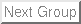Up: Lab 7: Sequential Circuits Previous: 2 Lab

Subsections

# 3 Supplementary Material

## 3.1 Verilog

### 3.1.1 Sequential HDL

So far the statement you have been using that are encapsulated by always where blocking type, i.e., they are executed sequentially. For this lab you will need to use a nonblocking procedural assignment. Nonblocking assignments are executed concurrently. To differentiate between the two lets look at the following two examples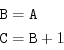(1)

and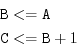(2)

The first case is the blocking one, the statements are executed sequentially, therefore the first statement stores A into B, then 1 is added to B and stored into C. At then end a value of A+1 is stored into C. On the other hand, the second case is nonblocking. In this case the assignments are made using <= sign instead of = sign. Nonblocking statements are executed concurrently, so values of the right hand side are stored in temporary location till the entire block is finished and then they will be assigned to the variables on the left. For this case C will contain the original value of B+1 rather than A+1 as in the first case.

Use blocking assignments when you must have a sequential execution. If you are modeling edge-sensitive behavior use nonblocking assignments

In synchronous sequential circuits, changes occur based on transitions rather than levels. In this case we need to indicate whether the change should occur based on the rising edge or the falling edge of the signal. This behavior is modeled using the two keywords posedge and negedge to represent rising and falling edge, respectively. For example,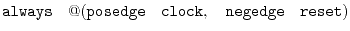(3)

will start executing on the rising edge of clock or on the falling edge of reset.

If your circuit has an asynchronous reset, so you may have an if-else statement to indicate whether you are resetting or triggering the circuit, in this case the very last statement of the nonblocking assignment needs to be the one related to the clock. For example for a D flip-flop with asynchronous reset you need to write it this way.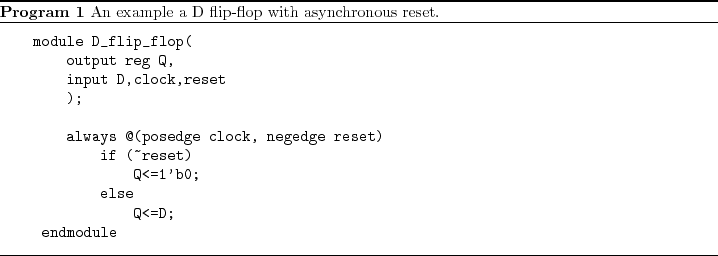Up: Lab 7: Sequential Circuits Previous: 2 Lab